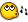# solviong angles

## Recommended Posts

here is a tiny script that helped mesolve my homework

```\$s = InputBox("angles", "type the number thats on your paper")
\$a = InputBox("angles","type the degree of the angle 90, 180, 360")
\$d = msgBox(0, "the answer is", \$a - \$s - \$s - \$s)
; to solve circle equations  add another - \$s and then divide the answer by 2
; where it says \$s = type whatever the number there is shown on your paper, \$a = whatever kind of angle it is 90, 180, 360.```

solving_angles__math_.au3

## Create an account

Register a new account

• ### Recently Browsing   0 members

×

• Wiki

• Back

• #### Beta

• Git
• FAQ
• Our Picks
×
• Create New...